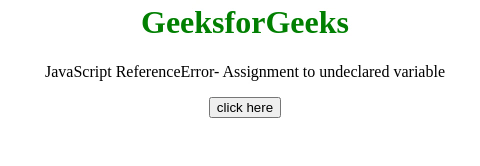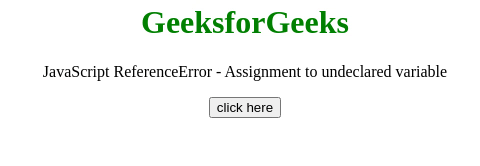Open in App
Not now

# JavaScript ReferenceError – Assignment to undeclared variable

• Last Updated : 24 Jul, 2020

This JavaScript exception Assignment to undeclared variable occurs in strict-mode If the value has been assigned to an undeclared variable.

Message:

```ReferenceError: assignment to undeclared variable "x" (Firefox)
ReferenceError: "x" is not defined (Chrome)
ReferenceError: Variable undefined in strict mode (Edge)
```

Error Type:

```ReferenceError
```

Cause of the error: Somewhere in the code, there is an assignment without the var, let or const keyword. When a value is assigned to an undeclared variable this error occurs.

Example 1: In this example, the const keyword is used with the variable assignment, So the error has not occurred.

## HTML

 ```<``html``>  ``<``head``>     ``   ``<``body` `style``=``"text-align:center;"``> ``    ``<``h1` `style``=``"color:green;"``>  ``        ``GeeksforGeeks  ``    `` ``     ` `    ``<``p``>``        ``JavaScript ReferenceError-``        ``Assignment to undeclared variable ``    ````    ` `    ``<``button` `onclick` `= ``"Geeks();"``>``        ``click here``    ````    ``<``p` `id``=``"GFG_DOWN"``> ``    ````        ` `    ``<``script``> ``        ``var el_down = document.getElementById("GFG_DOWN");``        ``function GFG() { ``          ``'use strict'; ``           ``const var_1 = "Value assigned without declaration"; ``        ``}``        ``function Geeks() { ``            ``try {``                ``GFG();``                ``el_down.innerHTML = ``                ``"'Assignment to undeclared variable'"+``                ``"error has not occurred";``            ``} catch(e) {``                ``el_down.innerHTML = ``                ``"'Assignment to undeclared variable'"+``                ``"error has occurred";``            ``}``        ``} ``    `` ``   ```

Output:Example 2: In this Example, the var, let or const keyword is not used with the variable assignment, So the error has occurred.

## HTML

 ```<``html``>  ``<``head``>     ``   ``<``body` `style``=``"text-align:center;"``> ``    ``<``h1` `style``=``"color:green;"``>  ``        ``GeeksforGeeks  ``    `` ``     ` `    ``<``p``>``        ``JavaScript ReferenceError -``        ``Assignment to undeclared variable ``    ````    ` `    ``<``button` `onclick` `= ``"Geeks();"``>``        ``click here``    ````    ``<``p` `id``=``"GFG_DOWN"``> ``    ````        ` `    ``<``script``> ``        ``var el_down = document.getElementById("GFG_DOWN");``        ``function GFG() { ``          ``'use strict'; ``          ``var_1 = true; ``        ``}``        ``function Geeks() { ``            ``try {``                ``GFG();``                ``el_down.innerHTML = ``                ``"'Assignment to undeclared variable'"+``                ``"error has not occurred";``            ``} catch(e) {``                ``el_down.innerHTML = ``                ``"'Assignment to undeclared variable'"+``                ``"error has occurred";``            ``}``        ``} ``    `` ``   ```

Output:My Personal Notes arrow_drop_up